Olympiad Test: Inserting the Missing Character

# Olympiad Test: Inserting the Missing Character

Test Description

## 15 Questions MCQ Test Science Olympiad Class 9 | Olympiad Test: Inserting the Missing Character

Olympiad Test: Inserting the Missing Character for Class 9 2022 is part of Science Olympiad Class 9 preparation. The Olympiad Test: Inserting the Missing Character questions and answers have been prepared according to the Class 9 exam syllabus.The Olympiad Test: Inserting the Missing Character MCQs are made for Class 9 2022 Exam. Find important definitions, questions, notes, meanings, examples, exercises, MCQs and online tests for Olympiad Test: Inserting the Missing Character below.
Solutions of Olympiad Test: Inserting the Missing Character questions in English are available as part of our Science Olympiad Class 9 for Class 9 & Olympiad Test: Inserting the Missing Character solutions in Hindi for Science Olympiad Class 9 course. Download more important topics, notes, lectures and mock test series for Class 9 Exam by signing up for free. Attempt Olympiad Test: Inserting the Missing Character | 15 questions in 15 minutes | Mock test for Class 9 preparation | Free important questions MCQ to study Science Olympiad Class 9 for Class 9 Exam | Download free PDF with solutions
 1 Crore+ students have signed up on EduRev. Have you?
Olympiad Test: Inserting the Missing Character - Question 1

### Direction: Find the value of (?) in the following question: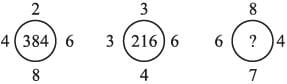Detailed Solution for Olympiad Test: Inserting the Missing Character - Question 1

Here, 4 × 2 × 8 × 6 = 384
3 ×  3 × 4 × 6 = 216
∴ 6 ×  8 × 7 × 4 = 1344

Olympiad Test: Inserting the Missing Character - Question 2

### Direction: Find the value of (?) in the following question: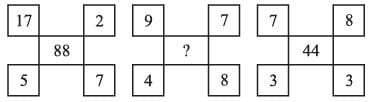Detailed Solution for Olympiad Test: Inserting the Missing Character - Question 2

Here, (17 – 5) × (2 + 7) = 12 × 9 = 108
and (7 – 3) × (8 + 3) = 4 × 11 = 44
∴ ? =  (9 – 4) × (7 + 8) = 5 × 15 = 75

Olympiad Test: Inserting the Missing Character - Question 3

### Direction: Find the value of (?) in the following question: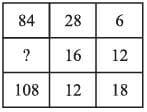Detailed Solution for Olympiad Test: Inserting the Missing Character - Question 3

Here, 28 ÷ 2 × 6 = 14 × 6 = 84
∴ 12 ÷ 2 × 18 = 6 × 18 = 108
16 ÷ 2 × 12 = 8 × 12 = 96

Olympiad Test: Inserting the Missing Character - Question 4

Direction: Find the value of (?) in the following question: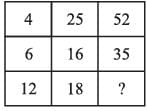Detailed Solution for Olympiad Test: Inserting the Missing Character - Question 4

In 1st row, (25 × 2) + (4 ÷ 2) = 52
In 2nd row, (16 × 2) + (6 ÷ 2) = 35
In 3rd row, (18 × 2) + (12 ÷ 2) = 42

Olympiad Test: Inserting the Missing Character - Question 5

Direction: Find the value of (?) in the following question: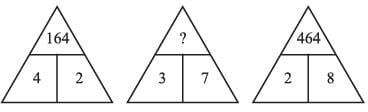Detailed Solution for Olympiad Test: Inserting the Missing Character - Question 5

In I figure 42 → 42 22 → 164
In III figure 28 → 22 82 → 464
∴ 37 →  32 72 → 949

Olympiad Test: Inserting the Missing Character - Question 6

Direction: In each of the following questions a set of figures carrying certain characters is given. The characters in each set follow a similar pattern. What is the missing character in each case?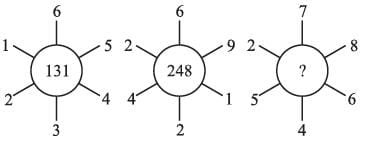Detailed Solution for Olympiad Test: Inserting the Missing Character - Question 6

In figure I, 2 – 1 = 1, 6 – 3 = 3, 5 – 4 = 1 ⇒ 131
In figure II, 4 – 2 = 2, 6 – 2 = 4, 9 – 1 = 8 ⇒ 248
In figure III, 5 – 3 = 3, 7 – 4 = 3, 8 – 6 = 2 ⇒ ? = 332

Olympiad Test: Inserting the Missing Character - Question 7

Direction: In each of the following question a set of figures carrying certain characters is given. The characters in each set follow a similar pattern. What is the missing character in each case?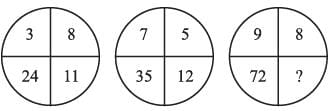Detailed Solution for Olympiad Test: Inserting the Missing Character - Question 7

In figure I, 24 ÷ 3 = 8 ⇒ 8 + 3 = 11
In figure II, 35 ÷ 7 = 5 ⇒ 5 + 7 = 12
In figure III, 72 ÷ 9 = 8 ⇒ 8 + 9 = 17

Olympiad Test: Inserting the Missing Character - Question 8

Direction: In each of the following question a set of figures carrying certain characters is given. The characters in each set follow a similar pattern. What is the missing character in each case?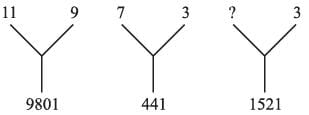Detailed Solution for Olympiad Test: Inserting the Missing Character - Question 8

In figure I, 112 × 92 = 121 × 81 = 9801
In figure II, 72 × 32 = 49 × 9 = 441
In figure III, x2 × 32 = 1521
⇒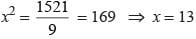Olympiad Test: Inserting the Missing Character - Question 9

Direction: In each of the following question a set of figures carrying certain characters is given. The characters in each set follow a similar pattern. What is the missing character in each case?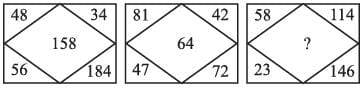Detailed Solution for Olympiad Test: Inserting the Missing Character - Question 9

In figure I, (184 – 34) + (56 – 48) = 150 + 8 = 158
In figure II, (72 – 42) + (81 – 47) = 30 + 34 = 64
In figure III, (146 – 114) + (58 – 23) = 32 + 35 = 67

Olympiad Test: Inserting the Missing Character - Question 10

Direction: In each of the following question a set of figures carrying certain characters is given. The characters in each set follow a similar pattern. What is the missing character in each case?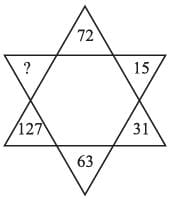Detailed Solution for Olympiad Test: Inserting the Missing Character - Question 10

Here, 7 × 2 + 1 = 15; 15 × 2 + 1 = 31;
31 × 2 + 1 = 63; 63 × 2 + 1 = 127;
∴ ? = 127 × 2 + 1 = 255

Olympiad Test: Inserting the Missing Character - Question 11

Direction: In each of the following question a set of figures carrying certain characters is given. The characters in each set follow a similar pattern. What is the missing character in each case?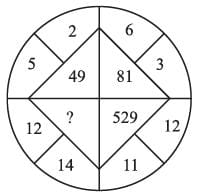Detailed Solution for Olympiad Test: Inserting the Missing Character - Question 11

In I part, 5 + 2 = 7 → 72 = 49
In II part, 6 + 3 = 9 → 92 = 81
In III part, 11 + 12 = 23 → 232 = 529
In IV part, 12 + 14 = 26 → 262 = 676

Olympiad Test: Inserting the Missing Character - Question 12

Direction: In each of the following question a set of figures carrying certain characters is given. The characters in each set follow a similar pattern. What is the missing character in each case?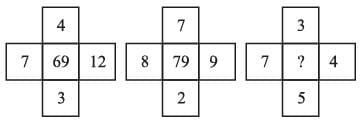Detailed Solution for Olympiad Test: Inserting the Missing Character - Question 12

In figure I, (7 × 3 ) + (4 × 12) = 21 + 48 = 69
In figure II, (8 × 2) + (7 × 9) = 16 + 63 = 79
In figure III, (7 × 5) + (3 × 4) = 35 + 12 = 47

Olympiad Test: Inserting the Missing Character - Question 13

Direction: In each of the following question a set of figures carrying certain characters is given. The characters in each set follow a similar pattern. What is the missing character in each case?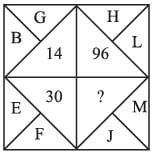Detailed Solution for Olympiad Test: Inserting the Missing Character - Question 13

Here, BG → 2 × 7 = 14
EF → 5 × 6 = 30
HL → 8 × 12 = 96
∴ ? = JM → 10 × 13 = 130

Olympiad Test: Inserting the Missing Character - Question 14

Direction: In each of the following question a set of figures carrying certain characters is given. The characters in each set follow a similar pattern. What is the missing character in each case?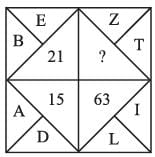Detailed Solution for Olympiad Test: Inserting the Missing Character - Question 14

Here, AD → 42 – 12 = 16 – 1 = 15
BE → 52 – 22 = 25 – 4 = 21
LI → 122 – 92 = 144 – 81 = 63
ZT → 262 – 202 = 676 – 400 = 276

Olympiad Test: Inserting the Missing Character - Question 15

Direction: In each of the following question a set of figures carrying certain characters is given. The characters in each set follow a similar pattern. What is the missing character in each case?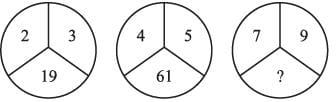Detailed Solution for Olympiad Test: Inserting the Missing Character - Question 15

In figure I, 33 – 23 = 27 – 8 = 19
In figure II, 53 – 43 = 125 – 64 = 61
In figure III, 93 – 73 = 729 – 343 = 386

## Science Olympiad Class 9

17 videos|6 docs|55 tests
 Use Code STAYHOME200 and get INR 200 additional OFF Use Coupon Code
Information about Olympiad Test: Inserting the Missing Character Page
In this test you can find the Exam questions for Olympiad Test: Inserting the Missing Character solved & explained in the simplest way possible. Besides giving Questions and answers for Olympiad Test: Inserting the Missing Character, EduRev gives you an ample number of Online tests for practice

## Science Olympiad Class 9

17 videos|6 docs|55 tests